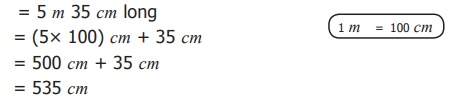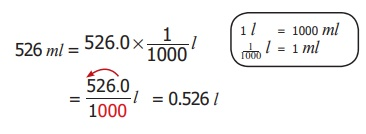Home | | Maths 6th Std | Conversions within the Metric System

# Conversions within the Metric System

All units of length in the metric system are defined in terms of the metre. A prefix is added to indicate the decimal place value position of the measurements.

Conversions within the Metric System:

All units of length in the metric system are defined in terms of the metre. A prefix is added to indicate the decimal place value position of the measurements. Similarly the units of weight and volume are defined in terms of gram and litre respectively. Let us observe the conversion chart.* when we move from higher unit to lower unit, multiply the given measure by the powers of 10's.

* when we move from lower unit to higher unit, divide the given measure by the powers of 10's.

Remember the following Conversion table:Before we study Conversion of metric units, we should learn about decimal point position when multiplying / dividing of a decimal number by the powers of ten.Example 1:

The official distance of Marathon race is 42.195 km. Express this distance in metre.

Solution : The official distance of Marathon race is 42.195 km.= 42.195 X 1000 m

= 42195 m

1 km = 1000 m

Example 2: The average rainfall of Tamilnadu is 998 mm. convert it into cm.

Solution :The average rainfall = 998 mm= 99.8 cm

Example 3:

A flag pole is 5 m 35 cm long. What is the length of the flag pole in cm?

Solution: The length of a flag pole = 5 m 35 cm long

1 m = 100 cm

= (5 ×  100) cm + 35 cm= 500 cm + 35 cm

= 535 cm

The flag pole is 535 cm long.

Example 4:

Janaki bought 650 mg of a tablet. What is its weight in gram?

Solution : weight of a tablet = 650 mgExample 5:

Murali has a bag that weighs 3 kg and 450 g.What is its weight in gram?

Solution: weight of Murali’s bag = 3 kg and 450 g ,

1 kg = 1000 g

= (3 × 1000 g) + 450 g

= 3000 g + 450 g

= 3450 g

Example 6:

A calf drinks 5.750 l of water ,convert into ml

Solution: Quantity of water drunk by the calf = 5.750 l= 5750 ml

Example 7: Convert 526 ml into l

Solution : 526 ml = 526.0 × (1/1000) lTry these

Convert the following

i) 23 km into m

23 km = 23 × 1000 = 23000 m

ii) 1.78 m into cm

1.78 m = 1.78 × 100 cm = 178 cm

iii) 7814 m into km

7814 m = 7814 ÷ 1000 km = 7.814 km

iv) 8.67 mm into cm

8.67 mm = 8.67 ÷ 10 cm = 0.867 cm

v) 16 l into ml

16 l = 16 × 1000 ml = 16000 ml

vi) 1500 ml into l

1500 ml = 1500 ÷ 1000 l = 1.500 l

vii) 2360 l into kl

2360l = 2360 ÷ 1000 kl = 2.360 kl

viii) 873 l into ml

873 l = 873 × 1000 ml = 873000 ml

ix) 40 mg into g

40 mg = 40 ÷ 1000 g = 0.040 g

x) 1550g into kg

1550 g = 1550 ÷ 1000 kg = 1.550 kg

xi) 6.5 kg into g

6.5 kg = 6.5 × 1000 g = 6500 g

xii) 723g into mg

723 g = 723 × 1000 mg  = 723000 mg

Think

Which of these is heavier in weight? 5 kg of cotton; 5000 g of Iron

Some measures that are not part of metric system.

1 inch = 2.54 cm

1 m = 3.281 ft

1 m = 39.37 inches

1 ft = 0.305 m = 30.48 cm

1 mile = 1.609 km

1 tonne = 1000 kg;

1 quintal = 100 kg;

1 tonne = 10 quintal

1 soverign = 8 g

TMC: (Thousand Million Cubic feet), 1 TMC =28,316,846,592 litres

1 kg = 1000 g

¼ kg = 250 g

½ kg = 500 g

¾ kg = 750 g

The following Tamil puzzle is taken from the famous Mathematics problems collection book in Tamil called “Kanakkathikaram” in which is a good example of conversion of units of distance :

முப்பத்தி ரணடு முழம்உளமுட் பனையை

தப்பாமல் ஒந்தி தவழ்ந்தேறிச் செப்பமுடச்

சாணேறி நான்குவிரற்கிழியும் என்பரே

நாணா தொரு நாள் நகர்ந்து

Meaning of this puzzle:

On a palm tree of height 32 cubit , a chameleon tried to reach the top of the tree. If it climbed one hand span on it but slipped four fingers on one day, in how many days will it reach the top of the tree?

Solution:

1 hand span = 12 fingers;

1 cubit = 2 hand spans = 24 fingers.

Height of the palm tree = 32 cubit = 32 × 24 fingers = 768 fingers.

Distance climbed in 1 day = 1 hand span = 12 fingers.

Distance slipped in 1 day = 4 fingers.

Actual distance climbed in 1 day = 12 − 4 = 8 fingers.

Number of days required to climb the top of the tree = 768/ 8 = 96 days

Tags : Measurements | Term 2 Chapter 2 | 6th Maths , 6th Maths : Term 2 Unit 2 : Measurements
Study Material, Lecturing Notes, Assignment, Reference, Wiki description explanation, brief detail
6th Maths : Term 2 Unit 2 : Measurements : Conversions within the Metric System | Measurements | Term 2 Chapter 2 | 6th Maths